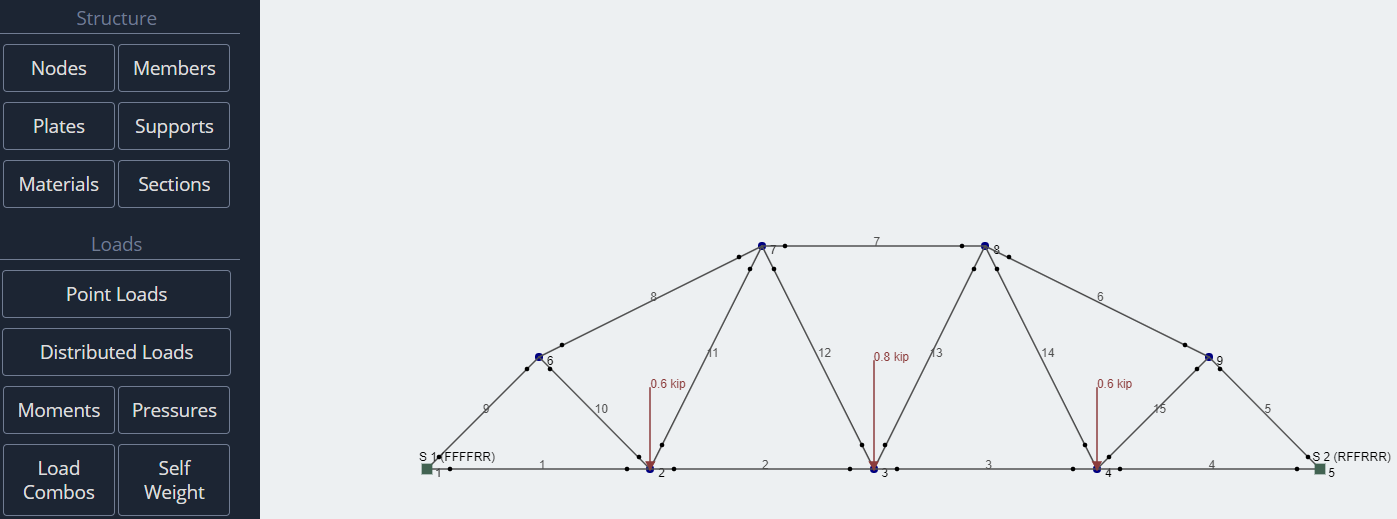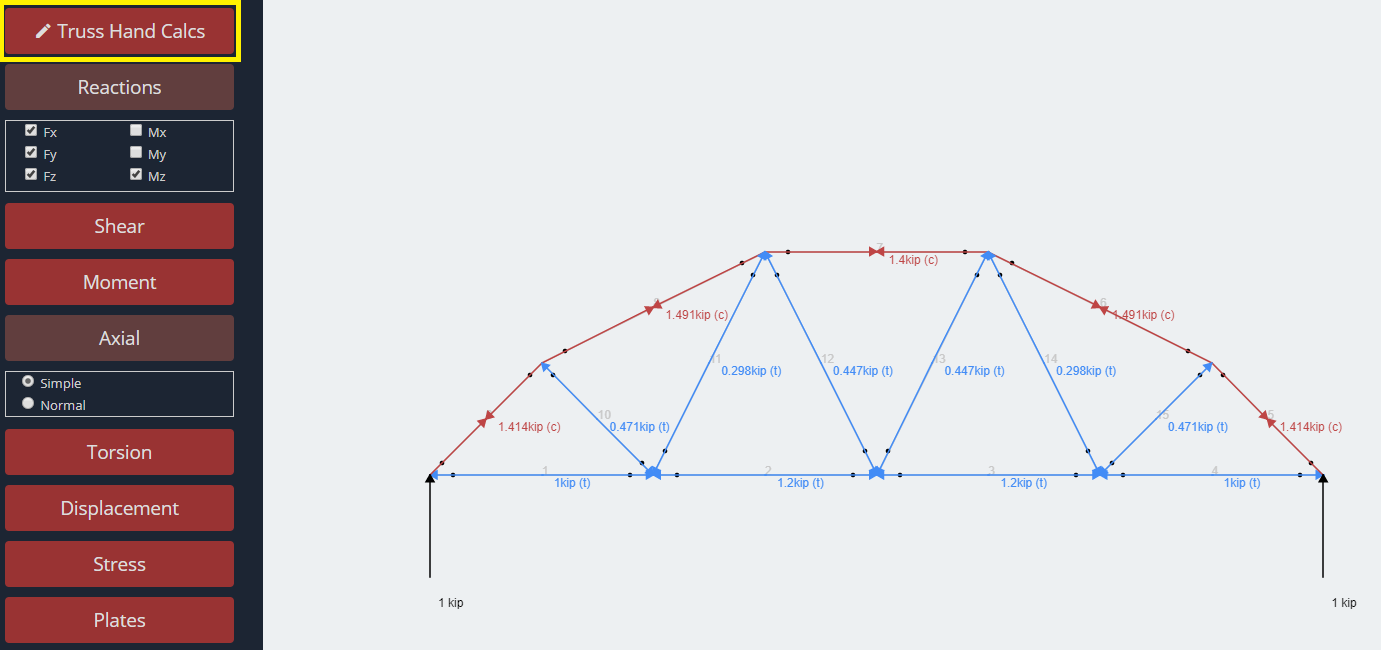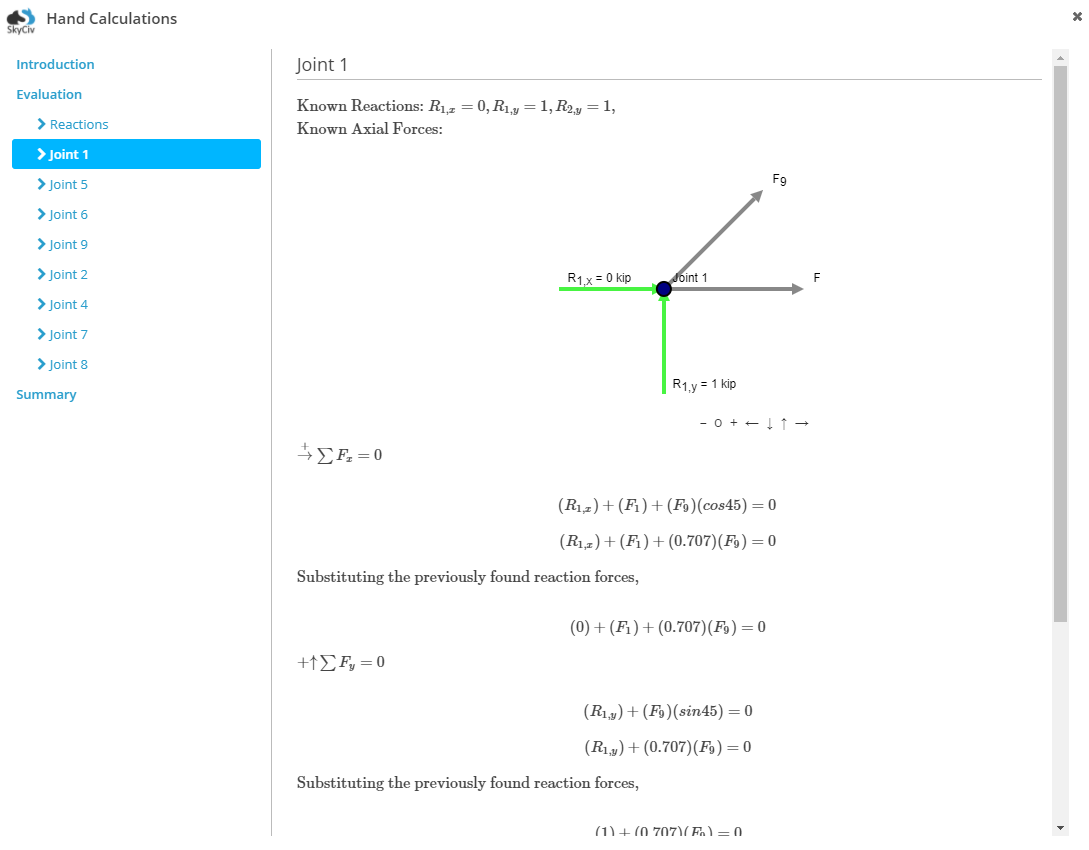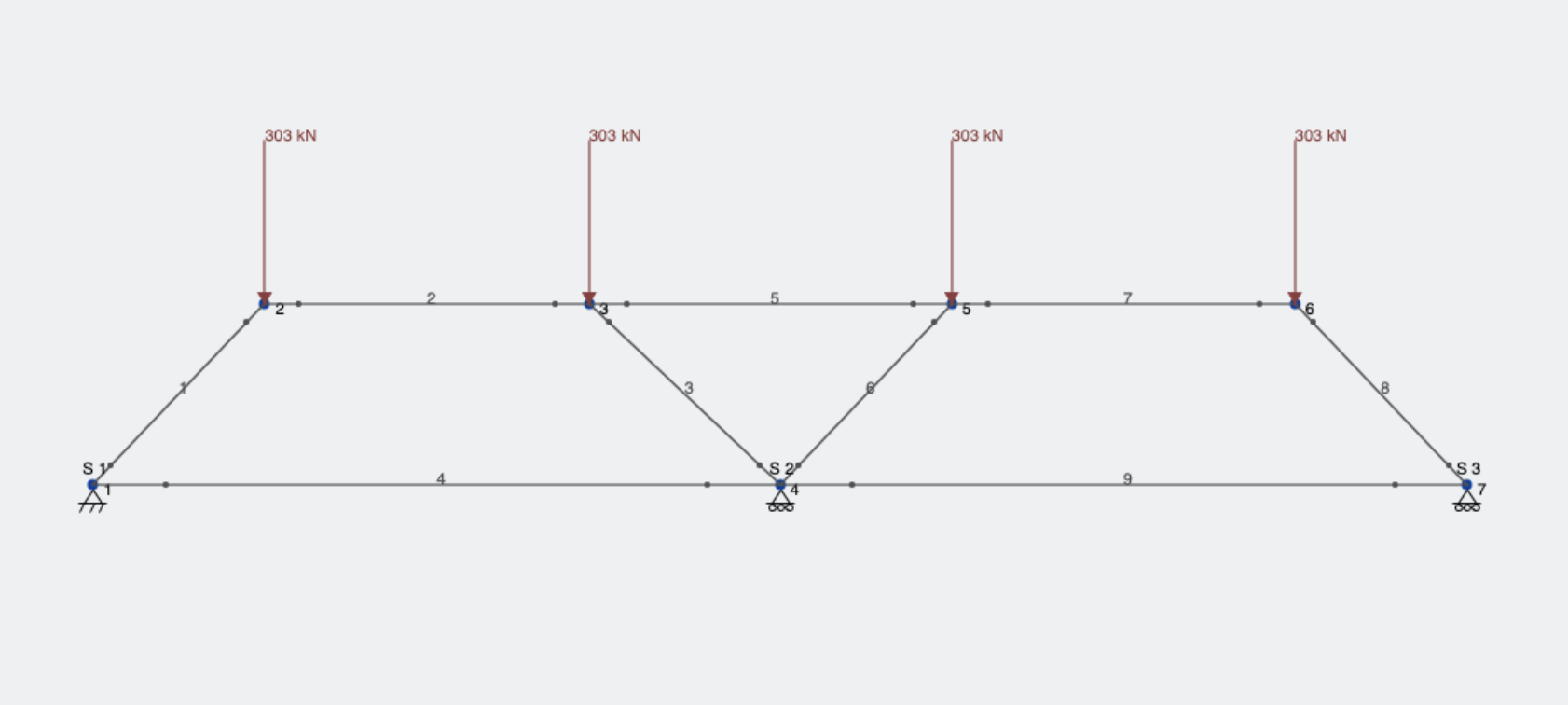SkyCiv Documentation

Your guide to SkyCiv software - tutorials, how-to guides and technical articles

1. Home
2. SkyCiv Structural 3D
3. Post Processing
4. Truss Hand Calculations

# Truss Hand Calculations

Truss hand calculations via the method of joints are available in the results section.

Reaction forces will first be attempted by analyzing the free body diagram (FBD) of the overall structure. Joints (aka nodes) are then analyzed via FBD’s to determine any axial forces (or previously unsolved reaction forces). This process continues across the joints until all axial forces have been solved. The following assumptions are made:

• All members are connected by pins. This implies that the member fixities are “FFFFRR” or “FFFFFR”
• All members are two force members and thus only support axial loads. This implies that only point loads that are applied to the nodes are allowed.

If either of the above conditions are not met, then the “Hand Calculation” button will not show

In addition, it should be noted that:

• Statically indeterminate members cannot be fully solved by the “Method of Joints” however, the hand calculations will still attempt as many solutions as possible for reactions and axial forces.
• All axial forces will be assumed to be in tension. This means that all positive results will confirm tension, negative results will determine compression, and zero results will determine 0 force members.
• In some cases, the “Method of Joints” is unable to provide a solution, and this is a limitation on the method. In these instances, the “Method of Sections” may be more appropriate.

# Example

1) Model your truss in S3D.2) Click “Solve” to transition to the results section. The reactions and axial buttons have been clicked on to show these results.3) Click the “Truss Hand Calcs” button highlighted in the image. Note that the button will only show if the members have fixities “FFFFRR” or “FFFFFR” representing pin fixities, and the structure is drawn in the x-y plane (i.e. all nodes must have the same z value).4) A pop-up will come up with the hand calculations. On the left is a menu you can click through to go through the introduction, evaluation of your structure, (if there are any issues with stability or determinacy), and then the free body diagrams and equilibrium equations for reactions and joints. The final summary menu item will provide a summary of all the axial and reaction results. Note, if the “Summary” menu does not appear, it means there were issues with completely solving your structure. This is likely due to the structure being statically indeterminate. In these cases, the hand calculations will still work, however not all axial forces will be found.

## Truss Instability Formula

The software can only solve structures that are stable. A helpful check for stability, are the following formulas:

• The condition m >= 2j – 3 must be satisfied for internal stability
• The condition m + r >= 2j must be satisfied for external stability

Where;
m = number of members
j = number of joints
r = number of reactions

Note: These calculations are made in the SkyCiv Truss software in the above Hand Calculation Module

So for example, in the following structure:We have 9 members, 7 joints and 3 reactions. This fails both the internal and external stability check criterion:

9 is not greater than 2(7) – 3 = 11 for internal stability
(9 + 3) is less than 2(7)  for external stability